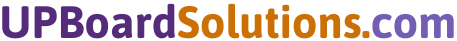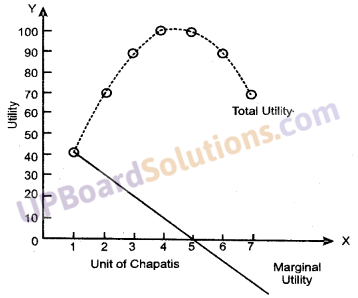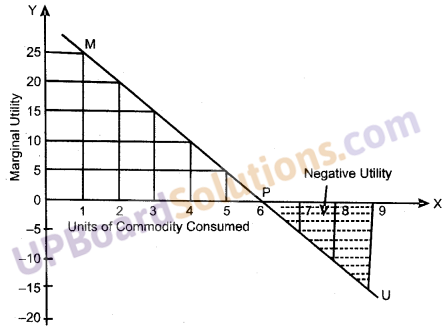# UP Board Solutions for Class 10 Commerce Chapter 18 Utility, Marginal Utility, Total Utility

## UP Board Solutions for Class 10 Commerce Chapter 18 Utility, Marginal Utility, Total Utility

Utility, Marginal Utility, Total Utility Objective Type Questions (1 Mark)

Question 1.
Marginal Utility may be:
(a) Positive
(b) Negative
(c) Zero
(d) All of these
(d) All of these

Question 2.
When the consumption of marginal unit gives neither satisfaction nor dissatisfaction, the marginal utility is:
(a) Positive
(b) Negative
(c) Zero
(d) All of these
(c) Zero

Question 3.
Marginal utility ………….. with successive units of consumption.
(a) Increases
(b) Decreases
(c) Either (a) or (b)
(d) None of these
(b) DecreasesQuestion 4.
Marginal utility is the ………… associated with the consumption of successive units.
(a) Sum of Marginal Utility
(b) Sumof Total Utility
(c) Neither (a) or (b)
(d) None of these
(a) Sum of Marginal Utility

Question 5.
When marginal utility diministes with successive units of consumption, the total utility at first increases at a ………. rate.
(a) Increasing
(b) Diminishing
(c) Decreasing
(d) None of these
(b) Diminishing

Utility, Marginal Utility, Total Utility Definite Answer Type Questions (1 Mark)

Question 1.
Who says that “the additional benefit which a person derives from a given increase of his stock of a thing diminishes with every increase in the stock that he already has.”
Prof. Marshall.Question 2.
What marginal is said when the consumption of the last unit gives some satisfaction?
Positive.

Utility, Marginal Utility, Total Utility Very Short Answer Type Questions (2 Marks)

Question 1.
Define Utility.
In common language, the word ‘utility’ means usefulness but in economics, it is used for the propriety in a commodity to satisfy human wants. According to Baugh, “Utility is (UPBoardSolutions.com) the capacity to satisfy human wants.”

Question 2.
Write two characteristics of utility.
The characteristics of the utility are as follows:

• Utility is created by Wants: Utility in any commodity is created by an effective desire to possess it. If a thing is not wanted, it has no utility.
• Utility is an abstract quality: Utility is (UPBoardSolutions.com) a commodity can only be experienced. It is a psychological characteristic. It cannot be seen or touched physically.

Question 3.
What are the two kinds of utility? (UP 2016)
Utility is of two kinds:

• Marginal Utility
• Total Utility.Utility, Marginal Utility, Total Utility Short Answer Type Questions (4 Marks)

Question 1.
What is the total utility?
Total Utility: The total utility from the consumption of an article is the sum of utilities of all units consumed at a particular time to satisfy a particular want. Prof. A. L. Meyers defines it as, “the sum of (UPBoardSolutions.com) the marginal utility associated with the consumption of the successive units.” Prof. Marshall says that “the total utility or the total pleasure or another benefit it yields him increases but does not increase at the same route as his stock increases.”

Question 2.
How marginal utility and total utility-related?
The relation between the total utility and marginal utility is deep. The total utility increases so long as the marginal utility is positive. The total utility is maximum when the marginal utility is zero. After this point, the total (UPBoardSolutions.com) utility begins to decrease and marginal utility is negative.

Question 3.
Distinguish between marginal utility and total utility. (UP 2019)

 Total Utility Marginal Utility 1. Meaning Total utility means total benefits obtained by a person from the consumption of goods and services. Marginal utility means the amount of utility a person gains from the consumption of each successive unit of a commodity. 2. Result Suffers from diminishing returns. Declines for each additional unit consumed.

Utility, Marginal Utility, Total Utility Long Answer Type Questions (8 Marks)

Question 1.
What do you mean by the total utility and marginal utility? Explain with the help of example and diagram. (UP 2008, 09)
Or
What do you understand by the term Total Utility and Marginal Utility? What are the kinds of Marginal Utility?
Total Utility: Total Utility is the sum of the utility derived from the (UPBoardSolutions.com) consumption of all the units of a commodity at the disposal of the consumer at a particular time.Marginal Utility: Marginal utility is the utility of the last unit of the commodity that is consumed at any particular time. In order to satisfy our wants, we have to consume a number of units of a commodity. For instance, a person consumes 2 bananas yielding 6 and 4 units of utility at a time, the marginal utility is the utility of second banana which is equal to 4. Now, further suppose that he takes one more banana yielding him utility, say 2 units, then in such a case third banana is the marginal unit and its marginal utility will be equal to 2.

The marginal utility may be of three kinds i.e., (i) positive, (ii) negative, and (iii) zero.

• Marginal utility is positive when the consumption of the last unit gives some satisfaction.
• Marginal utility is negative when the consumption of marginal unit gives some dissatisfaction or does not yield any utility.
• Marginal utility is zero when consumption neither provides (UPBoardSolutions.com) satisfaction nor causes any dissatisfaction.

Relationship between marginal and total utility

 Units of Chapatis consumed in succession Marginal Utility Remarks Units of Total Utility 1 40 Positive Marginal Utility 40 2 30 70 3 20 90 4 10 5 0 Zero Marginal Utility 100 6 -10 Negative Marginal Utility 100 7 -20 70

Marginal utility of a commodity to a person is based on two things. These two things are as follows :

• Consumption should be regular and unbroken.
• The utility in question should be the utility of the final unit (or marginal unit) consumed.

For example, if an individual takes only 3 units of chapatis and his hunger is satisfied then the marginal unit is the third one and the marginal utility is the utility derived from its consumption, i.e., 20 units as shown in the table.The relation between the total utility and marginal utility is deep. The total utility increases so long as the marginal utility is positive. The total utility is maximum when the marginal utility is zero. After this point, the (UPBoardSolutions.com) total utility begins to decrease and marginal utility is negative. This relationship between total and marginal utility is well explained through the following figure:
It can be exhibited with the help of the diagram given in figure.Question 2.
List the importance of Law of Diminishing Marginal Utility. (UP 2009)
The law of diminishing marginal utility is considered as one of the basic laws of Economics.
The importance of the law can be explained as under:
1. Basis of the Law of Demand: The law of diminishing marginal utility provides the function for various laws of consumption. The law of demand which is a very important law of consumption is based on the law of diminishing marginal utility.

2. Basis of Equi-marginal Utility: The law of equity-marginal utility or the law of substitution is derived from the law of diminishing marginal utility. A consumer does not spend his total income on the purchase of one (UPBoardSolutions.com) commodity alone, instead, he spends it on different commodities in such a manner as to ensure that the marginal utility obtained from every commodity purchased is in the same relationship to price.3. Basis of Consumer’s Surplus: The utility derived by a consumer from each successive unit of a commodity goes on diminishing, though the price of the article remains unchanged. He stops his purchase at that point where the utility derived equals utility lost. Thus, the law of diminishing marginal utility is the basis of the concept of consumer’s surplus.

4. Indicates Relation between Standard of Living and Efficiency: The law of diminishing marginal utility helps us to understand the reason for a fall in the efficiency owing to a rise in the standard of living of the people.

5. Importance in the Field of Production: The law of substitution is based on the law of diminishing marginal utility. Accordingly, the factors of production can be substituted. The process of substitution is influenced by the prevailing prices of the factor of production. The dearer factors will be substituted by cheaper factors. The law of diminishing marginal utility will prove helpful to understand the law of substitution.

6. Theory of Taxation and Public Expenditure: Prof. Marshall utilized the law of diminishing marginal utility to build up his theory of taxation and public expenditure.

7. Public Finance: Modern governments follow the system of taxation so as to transfer the purchasing power from the richer sections of the society to the poorer section. This will help to achieve greater economic equity among the various sections of the society. Taxation and public expenditure is undertaken by the government are based on the law of diminishing marginal utility.

8. Importance in the Field of Exchange: When the supply of any commodity (UPBoardSolutions.com) increases, its marginal utility decreases and the prices also come down. The commodities which are supplied largely have no value-in-exchange. Thus, the law has considerable importance in the field of exchange.Question 3.
What are the assumptions of Law of Diminishing Marginal Utility? (UP 2009)
The law of diminishing marginal utility is based on certain assumptions and any change in the assumptions would obstruct the operation of the Law. ‘Other things remaining the same’ signifies certain assumptions and conditions without which the law cannot operate. The law of diminishing marginal utility is based on the following important conditions or assumptions:

1. The Units of the Commodity must be Homogeneous: The first condition to be satisfied for the operation of the law is that units of the commodity must be similar in quality and quantity. Suppose, you give two oranges to (UPBoardSolutions.com) a hungry man to appease his hunger. The first orange which you gave him is a slightly damaged one while the second orange is a better one. Naturally, when the first orange was not tasty, he will derive more satisfaction from the second orange.Suppose, you give a third orange also, that would be the best of the three oranges; he may even derive more utility from its consumption than the other two oranges. If the units of the commodity are not similar in quality, the law of diminishing marginal utility will not operate. Similarly, the quantity of each unit must remain the same. If the first orange is very small in size while the second orange is double the size of the first orange, the law of diminishing marginal utility does not operate. Thus, if the units of the commodity are not alike in quality and size, the law may not operate.

2. The Process of Consumption should be Continuous: The second condition is that the period of consumption should be continuous and there should not arise any time-gap between consumption of the two units of the commodity. For example, (UPBoardSolutions.com) a person eats one chapati at 8 O’clock in the morning, the second chapati at 9 O’clock in the morning and the third chapati at 10 a.m. The law of diminishing marginal utility does not apply here because the period over which consumption is spread is not continuous and sufficient time interval given will obstruct the operation of the law. If the second chapati is taken immediately after the first chapati, the utility of the later will diminish.

3. The Price of the Commodity and its Substitutes should Remain the Same:
The price of the commodity should remain unchanged during the process of consumption. For instance, if the price of an article has reduced considerably consumers may want it with increased intensity and the desire to get more and more of that article will increase its utility. Therefore, the law of diminishing marginal utility will not operate as far as the commodity in question becomes cheap and one wants it with increased intensity.

The price of the substitutes should also remain the same for the operation of the law. If the cost of an article comes down, the demand for it will increase and it is natural to increase its utility also. If the price of the substitute of an article goes up, people start consuming the article in question and the utility of the article goes up, comparatively to that of the substitute. Thus, the (UPBoardSolutions.com) unchangeableness of the price of the article in question and the substitute thereof is very important for the operation of the law of diminishing marginal utility.

4. Taste, Habit, Fashion and Income of the Consumer should remain Unchanged: The law of diminishing marginal utility operates only if the taste, habit and income of the consumer do not change, in those cases where the period of consumption is long. For example, the particular design of a shirt which is not in fashion may yield only less utility to a person, but if the same shirt comes in fashion, its utility is liable to increase.5. Mental Approach: The mental outlook of the consumer should remain the same. Normally, a person who eats 5 chapatis should feel satisfied, and if he is forced to eat more, he will yield zero utility and later on negative utility. Suppose, he takes some intoxicants after eating 5 chapatis, he craves for more chapatis and subsequently its utility will increase rather than diminishing. Under such a situation the law does not work.

Question 4.
Explain the Law of Diminishing Marginal Utility in detail. (UP 2008)
Or
Explain the Law of Diminishing Marginal Utility with the help of a diagram. (UP 2011, 13)
Law of Diminishing Marginal Utility: Law of Diminishing Marginal Utility states that as a person purchases more and more units of a commodity, its marginal utility diminishes. The more we have of a commodity, the less urgently we want its subsequent units and the utility of its succeeding units goes on diminishing. According to Prof. Marshall, “The additional benefit which a person derives from a given increase of the stock of a thing diminishes with every increase in the stock, which he already has.” This concept can be cleared from the following example:This example makes it clear that as a person gets more and more units of a commodity, the marginal utility derived from the successive units diminishes.

Tabular Representation: The following table illustrates the Law of Diminishing Marginal Utility as applied to the consumption of slices of bread:

 Units (Slices of bread) Marginal Utility (Units of satisfaction) Total Utility 1 25 25 2 23 48 3 19 67 4 15 82 5 10 92 6 0 92 7 -12 80 8 -20 60

Marginal utility of bread till the 5th unit is positive if the consumer proceeds further to consume the 6th unit, marginal unit drops down to zero. After the zero points, utility becomes negative i.e., the 7th and 8th units give negative utility.
Diagrammatic Representation: The Law of Diminishing Marginal Utility can (UPBoardSolutions.com) be represented diagrammatically. The above illustration can be better understood with the help of the following diagram:Along the OX-axis units of the commodity is represented and along the OY-axis marginal utility is measured. ‘MU’ is the marginal utility curve. This curve slopes downwards to the right because with every additional increase in the quantity of the commodity consumed, there is a decline in the marginal utility. At the 6th unit, the marginal utility drops down to zero. ‘P’ is (UPBoardSolutions.com) the point of satiety, and then it becomes negative. The dotted area below the OX-axis represents negative utility.